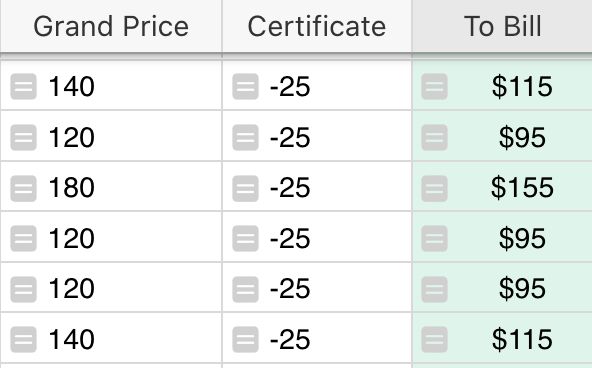# Sum a column and present the result

Hello all,
I have gone through the help and through the forum, but am unable to do a very simple task. I’d like to sum a column and present the sum on another widget. I tried a Summarisation table but didn’t get the info I needed.In the image above, the “To_Bill” column is the added sum of the total of “Grand_Total” and “Certificate”. I’d like the other table widget to show JUST the total of all the amounts (\$670) in the “To_Bill” column, Basically just like a Sum(A1:A6) in Excel would do.

Hi! Your guess is correct: summary tables is the right tool for this. Add a widget for this table, and click the 𝚺 icon to summarize the table, and then do NOT select any columns to group by. You will then get a single-row table with just the totals.

Thank you! Work brilliantly as always1 Like

Additionally to this question, is it possible to subtract two summary tables and place them into another table? E.G Take the “Billing Total” summary and minus the “PAYMENTS” summary and the result placed into “Remaining”.

@dmitry-grist are you able to assist with this?

Hi Leon!

At this time, summary tables can’t be called in formulas. This is something we would like to improve. In the meantime, we can use formulas to find the sums that are calculated in the summary tables then subtract the two to find the remaining amount. I created an example for you at the link below:

https://public.getgrist.com/rGhtxrAKCpKJ/Community-1152/m/fork

In the table ‘REMAINING’, I added a ‘Sum to Bill’ and ‘Sum Totals’ column so you can see that each part of the formula used in the remaining column does find the same value as the summary table for each.

The formula used to find the Sum To Bill is:

``````SUM(Billing_Total.lookupRecords().To_Bill)
``````

This formula looks up all records in the Billing Total table and pulls the value for each record from the To Bill column then uses SUM() to sum all of the values found.

The formula used to find the Sum Totals is:

``````SUM(Payments.lookupRecords().totals)
``````

This formula looks up all records in the Payments table and pulls the value for each record from the totals column then uses SUM() to sum all of the values found.

Now to find the Remaining value, we subtract the Payments value from the Billing Total value. Using the two formulas above, we can find the amount remaining.

The formula used to find the Remaining is:

``````SUM(Billing_Total.lookupRecords().To_Bill) - SUM(Payments.lookupRecords().totals)
``````

Let me know if you have any other questions1 Like

Hi @natalie-grist I really appreciate your feedback on this and a nice solution to the question. You guys rock!

1 Like